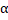# A uniform rodof mass 2 kg is hinged at one end.The rod is kept in the horizontal position by a massless string tied to point. Find the reaction of the hinge (in N) on endof the rod at the instant when string is cut## Question ID - 101435 :- A uniform rodof mass 2 kg is hinged at one end.The rod is kept in the horizontal position by a massless string tied to point. Find the reaction of the hinge (in N) on endof the rod at the instant when string is cut3537

(5)

When the string is cut, the weight of the rod constitutes torque about the hinge, so(i)

According to Newton's second law,(ii)

Whereis the angular acceleration of the rod about the end. From Eqs. (i) and (ii), we getOrHere, thereforeAcceleration of the CM of the rod isAgain by Newton’s second law,OrNext Question :

A point mass is tied to one end of a cord whose other end passes through a vertical hollow tube, caught in one hand. The point mass is being rotated in a horizontal circle of radius 2 m with a speed of 4 m/s. The cord is then pulled down so that the radius of the circle reduces to 1 m. Complete the ratio of kinetic energies under the final and initial states